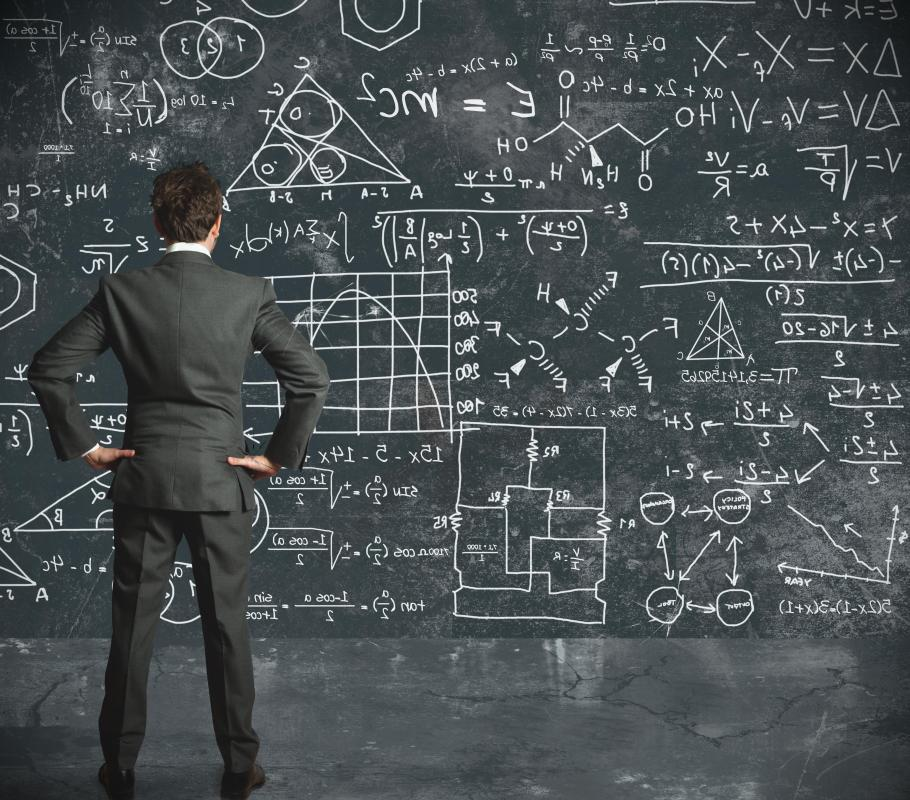# What is Kinetic Energy?

Phil Shepley
Phil Shepley

The extra energy that an object possesses when it is in motion is known as kinetic energy. This motion can be in any possible direction, and there are several different types of motion by which an object can move. Kinetic energy can also be described as the amount of work that it would require for the object to accelerate from a state of rest to its current velocity. The amount of this energy that an object may have is described simply as a magnitude, and does not represent its direction of travel.

The mathematical equation used to describe the kinetic energy of a non-rotating object is as follows:

KE = 1/2 * m * v&sup2Swinging on a swing is a good example of kinetic energy.

In the above equation, KE is the kinetic energy of the object, while m represents its mass and v its velocity, or speed. The resulting number one may arrive to is described in joules, which are the unit of work. What the equation says is that the kinetic energy of an object is directly proportional to the value of its squared speed. For example, if the speed of an object doubles, that means its kinetic energy will increase by four times as much; if the speed triples, it will increase by nine times, and so on.Kinematics is the study of motion and the development of equations to describe the motions of objects.

The previous equation described kinetic energy in terms of classical mechanics, meaning that the object is rigid and its motion is simplified. This type is known as translational motion, where an object simply moves from one point to another. There are other ways an object can move where computing its kinetic energy can be more complex, including vibrational motion and rotational motion. There are also instances when objects interact and can transfer this energy between one another.

Many objects simultaneously in motion have what is referred to as the kinetic energy of a system, where the total amount of energy is equal to the sum of the from each of the individual objects. The equations for calculating this energy become more complex with rotational and vibrational energy, and when there is a system of objects with various types of motion or non-rigid objects. Similarly, its calculation also becomes much more complicated when applied to quantum mechanics and other types of modern physics.

## You might also LikeJaniya

Yes that is pretty much the same thing.

anon159777

its useful but i don't get much information about what I'm looking for.

anon153220

This site is very useful.I found explanations of various terms related to physics in this site. And I understood them clearly. Thank you.

anon68154

no it's not, because a shotgun doesn't involve any kinetic energy. it is thermal energy and then it gets transferred to potential energy as it leaves the barrel of the gun. so there is your answer.

succulents

Is Kinetic energy the same thing as Potential energy?

I know that a shotgun blast has kinetic energy as a group that is more powerful than a single bullet, but does the same hold true for something like a flock of birds too? Or are the birds always counted as individuals?